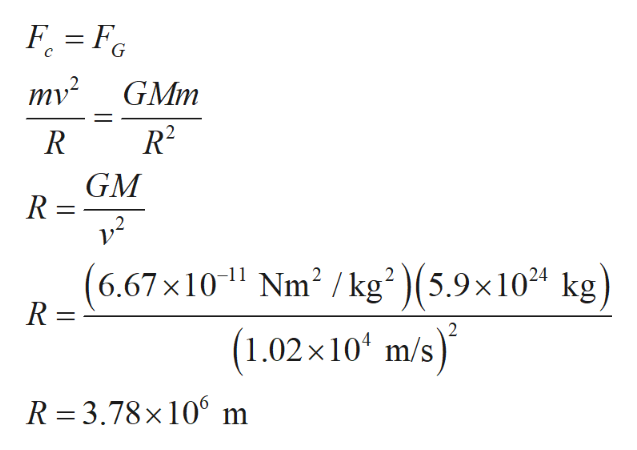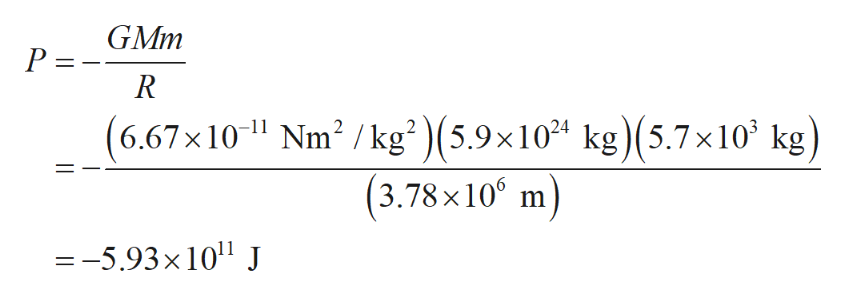# A satellite is traveling around a planet in a circular orbit with radius R. It moves in a constant speed of v = 1.02 × 104 m/s. The mass of the planet is M = 5.9 × 1024 kg. The mass of the satellite is m = 5.7 × 103 kg.a)Calculate the value of PE in joules.b)Enter an expression for the total energy E of the satellite in terms of m and v.c) Calculate the value of the total energy E in joules.

Question
23 views

A satellite is traveling around a planet in a circular orbit with radius R. It moves in a constant speed of v = 1.02 × 104 m/s. The mass of the planet is M = 5.9 × 1024 kg. The mass of the satellite is m = 5.7 × 103 kg.

a)Calculate the value of PE in joules.

b)Enter an expression for the total energy E of the satellite in terms of m and v.

c) Calculate the value of the total energy E in joules.

check_circle

Step 1

Consider a satellite having mass m orbiting around a planet in radius R with speed v and the planet’s mass is denoted by M.

Step 2

The needed centripetal force(Fc) is assisted by the gravitational force(FG). Find the radius(R) of the orbit by equation these two forces.help_outlineImage Transcriptionclosemv2 GMm R GM R = (6.67x1011 Nm2 /kg2 )(5.9x10 (1.02x104 m/s) kg) R = 2 R 3.78x 100 m fullscreen
Step 3

(a) write the expression for potential energy(P) and plug the requi...help_outlineImage TranscriptioncloseGMm P R (6.67x10 Nm2 /kg2 )(5.9x1024 kg)(5.7x10 kg) -11 (3.78 x100 m =-5.93x 10 J fullscreen

### Want to see the full answer?

See Solution

#### Want to see this answer and more?

Solutions are written by subject experts who are available 24/7. Questions are typically answered within 1 hour.*

See Solution
*Response times may vary by subject and question.
Tagged in
SciencePhysics

### Gravitation